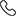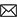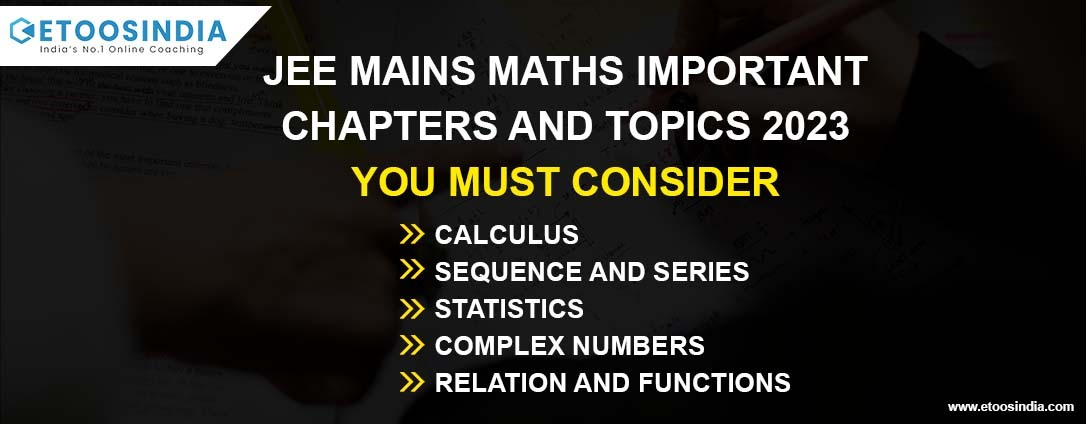Sales :9214233303 / Tech. Support Desk :9214233360/40 (9AM ~ 6PM)# JEE Mains Maths Important Chapters and Topics 2023 You Must Consider

25 Jan, 2021, By Tanvi SharmaJEE is known to be one of the toughest exams in the country. Every year there are lakhs of students who appear for the JEE mains exam but only a few are able to make through it. You need to be very determined towards your goal if you want to score well. The competition is really tough and the syllabus is also vast.

Completing the entire JEE syllabus once isn’t enough, the student needs to practice and revise as much as possible to get on the toppers’ list. The JEE paper includes questions from three subjects, namely physics, chemistry, and maths. The students often find it difficult to deal with the concepts of math. Here we have for the assistance of students, jee main math important topics.

## JEE Main Maths Important Chapters & Topics List

If you were fed-up with searching for the jee main math important topics then all that you need to do is follow our article and you will get all your answers. By analyzing the previous years question papers the ETOOSINDIA team has prepared the list of important chapters of math for jee mains.

So let's have a look at chapter wise jee mains maths important chapters.

Calculus- it is a combination of subtopics. If you are able to gain command over this topic then you can easily score 12-16 marks. Every year, in the JEE mains question paper 3-4 questions are put from this topic. You shouldn’t choose to skip this topic as it is really very important from an exam point of view. The subtopics of calculus are:

Differentiation- it is one of the important topics in maths for jee mains, but the students can score easily in this section.

Integration-it is one of the most difficult parts of calculus, as the variety of questions is high.

Limits, Continuity, and Differentiability-Another Maths Important Chapters is limits, continuity and differentiability. the questions asked from these topics are hard. It is a huge topic; the student needs to put sufficient time into it.Matrices and Determinants- if the students are able to follow the short tricks in the right manner, then the students can easily score good marks in this section. 2 questions are definitely asked from this chapter hence it is put in the category of Important Topics for JEE Mains 2023 Mathematics

Trigonometry-well, there is no surety about the number of questions appearing from this topic. So it can be put under-hit or miss type of topic. But the students need to understand the concepts of this topic properly as it’s related to the concepts of other topics also.

Sequence and Series- 1-2 questions are put from this topic every year. The topic is time-consuming and difficult but the student can’t ignore it as it is one of the most important topics.

Probability- again you need to give proper time to this topic as it is an important topic in math for jee mains and also the questions are typical. 1-2 questions are surely asked from this topic.

Sets and Functions- the students need to gain command over this topic to be able to understand the other topics as this one is related to other jee main 2023 maths important topics.

Statistics- it is an easy topic, the students can gain score in the question asked from this. Around 1-2 questions are asked from statistics. It is also one of the math important chapters for jee mains.

You need to have the best study material with you if you want to score well in the math section, you can get ETOOSINDIA study material. With the help of Etoosidnia, you will get to study from the top faculties of Kota.

You can get Etoosindia video lectures of the maths important chapters for jee mains, previous years' question papers, and JEE sample question papers. To help the students in making them understand the Important Topics for JEE Mains 2023 Mathematics doubts, the Etoos India have for the students live doubt session.

### JEE Mains Maths Important Chapters with Weightage 2023

If you are looking for the weightage of the topics that are a part of the syllabus, hence here we have the list for you. Get to know about the weightage and draft your JEE preparation strategies accordingly.

 Topic Name No of questions Coordinate Geometry 5 Limits, Continuity and Differentiability 3 Integral Calculus 3 Complex numbers and Quadratic Equation 2 Matrices and Determinants 2 Statistics and Probability 2 Three Dimensional Geometry 2 Vector Algebra 2 Sets, Relation and Function 1 Permutations and Combinations 1 Binomial Theorem and Its Application 1 Sequences and Series 1 Trigonometry 1 Mathematical Reasoning 1 Differential Equation 1 Statics and Dynamics 1 Differential Calculus 1

Keep following EtoosIndia JEE Articles for the latest updates.